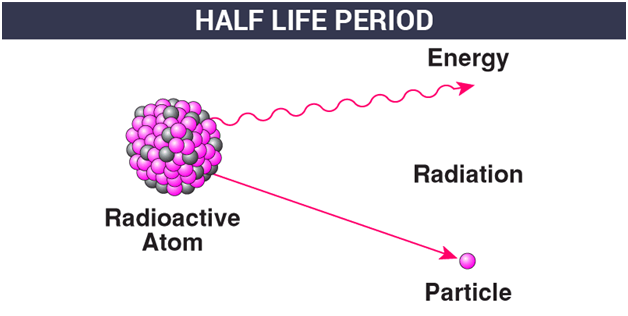# Half Life PeriodHalf-Life or previously known as Half-Life Period is one of the common terminologies used in Physics to describe the radioactive decay of a particular sample or element within a certain period of time. Students of nuclear Physics will come across the term often while studying the subject.

However, this concept is also widely used to describe various types of decay processes especially exponential and non-exponential decay. Apart from physics, the term is used in medical sciences to represent the biological half-life of certain chemicals in the human body or in drugs.

## Half-Life Definition

Half-Life is normally defined as the time needed by a radioactive substance (or one half the atoms) to disintegrate or transform into a different substance. The principle was first discovered in 1907 by Ernest Rutherford. It is usually represented by the symbol Ug or t1/2.

To help you understand the concept better if we take a radioactive element which has a one-hour half-life. In this case, the one half would decay within an hour and the remaining would decay within another hour. The question that arises here is, why the remaining material did not decay in the hour?

Well, if the radioactive element is taken in a case where half of the atoms have decayed after one-half life, it would be proper to assume that they have a life expectancy at the average level as well-defined viz. the atoms with mean life that is much longer than their half-life. This would mean that the half-life divided by 2 which is the natural algorithm would be the mean life. On the other hand, the half-life is often defined in terms of probability.

### Understanding The Concept Via Experiment

To know how radioactive decay happens, let us do an experiment with a fair cluster of people owing to the fact that the statistical observation would give a fairly clear outcome.

Let’s take a scenario, where close to 1000 people are inside a hall with each one among the group been given a coin. The coin would denote the capacity to decay and each individual represents the atom with radioactivity. The individuals could be asked to toss their coins per minute once.  If the result of the toss heads, the individual could be asked to leave the hall (denoting atom decay) and if the result is tails, there is no need to take action except waiting for one minute more for another attempt for the toss.

From the above example, you would have realized as to how this topic has been discussed with enthusiasm among students owing to its importance.  This is why the topic of radioactivity would be researched much extensively in the future in the years to come.

## Half-Life Formulas

Below students will find the formulas for half-life that are used to describe the decay in substances.

1. N(t) = No (½) t / t ½
2. N(t) = No e-t / r
3. N(t) = Noeλt

Here we consider the following,

• N0 = the initial quantity of the substance
• N(t) = the quantity that is left over
• t1⁄2 = half-life
• τ = mean lifetime of the decaying quantity
• λ = decay constant
Test your knowledge on Half Life Period